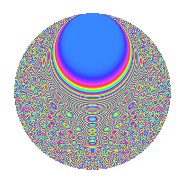# Properties

 Label 8013.2.aLevel 8013 Weight 2 Character orbit a Rep. character $$\chi_{8013}(1,\cdot)$$ Character field $$\Q$$ Dimension 445 Newform subspaces 4 Sturm bound 1781 Trace bound 1

# Related objects

## Defining parameters

 Level: $$N$$ = $$8013 = 3 \cdot 2671$$ Weight: $$k$$ = $$2$$ Character orbit: $$[\chi]$$ = 8013.a (trivial) Character field: $$\Q$$ Newform subspaces: $$4$$ Sturm bound: $$1781$$ Trace bound: $$1$$

## Dimensions

The following table gives the dimensions of various subspaces of $$M_{2}(\Gamma_0(8013))$$.

Total New Old
Modular forms 892 445 447
Cusp forms 889 445 444
Eisenstein series 3 0 3

The following table gives the dimensions of the cuspidal new subspaces with specified eigenvalues for the Atkin-Lehner operators and the Fricke involution.

$$3$$$$2671$$FrickeDim.
$$+$$$$+$$$$+$$$$116$$
$$+$$$$-$$$$-$$$$106$$
$$-$$$$+$$$$-$$$$129$$
$$-$$$$-$$$$+$$$$94$$
Plus space$$+$$$$210$$
Minus space$$-$$$$235$$

## Trace form

 $$445q + q^{2} + q^{3} + 449q^{4} - 2q^{5} + 3q^{6} + 8q^{7} + 9q^{8} + 445q^{9} + O(q^{10})$$ $$445q + q^{2} + q^{3} + 449q^{4} - 2q^{5} + 3q^{6} + 8q^{7} + 9q^{8} + 445q^{9} + 2q^{10} - q^{12} + 2q^{13} + 16q^{14} + 6q^{15} + 461q^{16} - 6q^{17} + q^{18} + 8q^{19} + 22q^{20} + 4q^{21} + 8q^{22} + 8q^{23} + 3q^{24} + 439q^{25} + 2q^{26} + q^{27} + 24q^{28} + 2q^{29} + 2q^{30} + 24q^{31} + 29q^{32} + 4q^{33} + 14q^{34} + 16q^{35} + 449q^{36} + 2q^{37} - 12q^{38} + 6q^{39} - 6q^{40} - 22q^{41} - 20q^{42} - 20q^{43} + 16q^{44} - 2q^{45} + 24q^{47} + 15q^{48} + 453q^{49} - 17q^{50} - 2q^{51} - 14q^{52} + 6q^{53} + 3q^{54} + 40q^{55} + 40q^{56} + 18q^{58} + 8q^{59} + 22q^{60} - 6q^{61} + 4q^{62} + 8q^{63} + 505q^{64} - 44q^{65} + 12q^{66} + 24q^{67} - 58q^{68} - 36q^{70} + 32q^{71} + 9q^{72} - 18q^{73} + 10q^{74} - 17q^{75} + 100q^{76} + 60q^{77} + 2q^{78} + 24q^{79} - 6q^{80} + 445q^{81} - 54q^{82} + 20q^{83} + 20q^{84} - 8q^{85} + 44q^{86} + 6q^{87} + 40q^{88} - 30q^{89} + 2q^{90} - 4q^{91} + 4q^{92} - 12q^{93} + 8q^{95} + 19q^{96} + 14q^{97} + 41q^{98} + O(q^{100})$$

## Decomposition of $$S_{2}^{\mathrm{new}}(\Gamma_0(8013))$$ into newform subspaces

Label Dim. $$A$$ Field CM Traces A-L signs $q$-expansion
$$a_2$$ $$a_3$$ $$a_5$$ $$a_7$$ 3 2671
8013.2.a.a $$94$$ $$63.984$$ None $$-13$$ $$94$$ $$-14$$ $$-55$$ $$-$$ $$-$$
8013.2.a.b $$106$$ $$63.984$$ None $$15$$ $$-106$$ $$16$$ $$35$$ $$+$$ $$-$$
8013.2.a.c $$116$$ $$63.984$$ None $$-16$$ $$-116$$ $$-20$$ $$-33$$ $$+$$ $$+$$
8013.2.a.d $$129$$ $$63.984$$ None $$15$$ $$129$$ $$16$$ $$61$$ $$-$$ $$+$$

## Decomposition of $$S_{2}^{\mathrm{old}}(\Gamma_0(8013))$$ into lower level spaces

$$S_{2}^{\mathrm{old}}(\Gamma_0(8013)) \cong$$ $$S_{2}^{\mathrm{new}}(\Gamma_0(2671))$$$$^{\oplus 2}$$

## Hecke Characteristic Polynomials

There are no characteristic polynomials of Hecke operators in the database